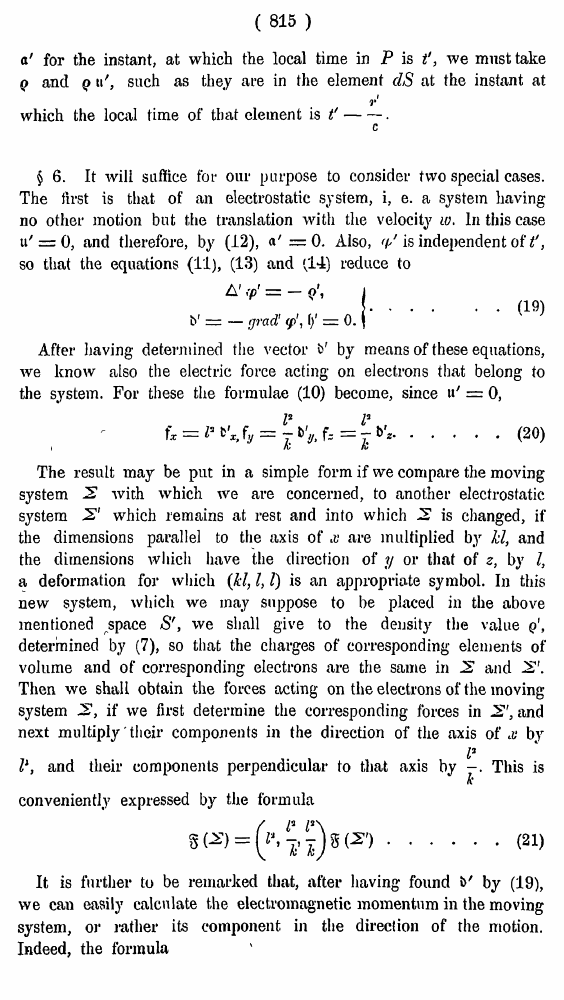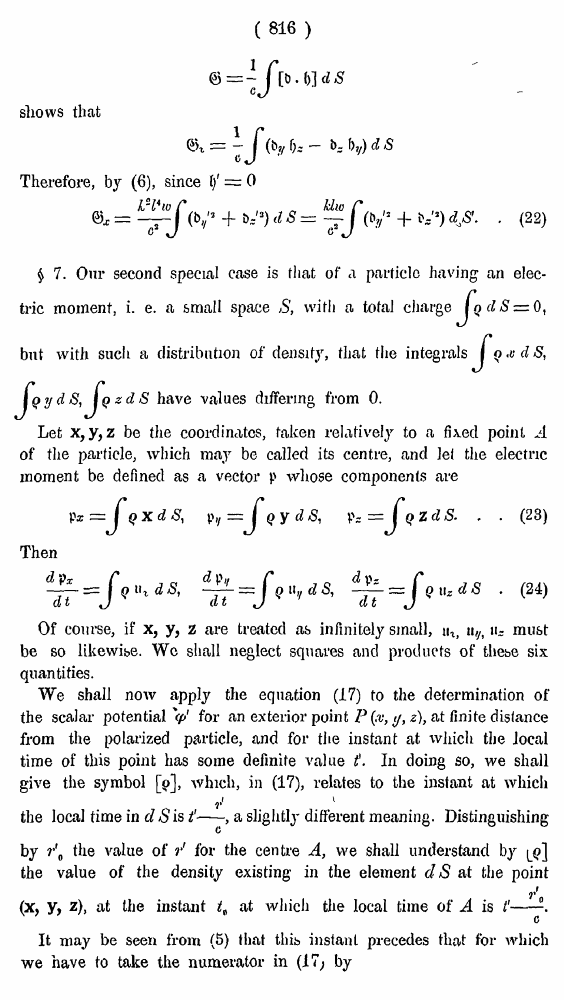# H. A. Lorentz. Electromagnetic phenomena in a system moving with any velocity smaller than that of light. // Proceedings Royal Acad., Amsterdam. Vol. VI., 1904

ô ÚÁ¼ÁŠŸ   áÞµÐÒÍ ¶ŸÞšÁ·«   <<<     î·ÞÁÚÒ—Á 815   >>>809  810  811  812  813  814  815 816  817  818  819  820  821  822  823  824  825  826  827  828  829  830  831 ( 815 ) a' for the instant, at which the local time in P is t', we must take q and q u', such as they are in the element clS at the instant at which the local time of that clement is Ï 6. It will suffice fot our purpose to consider two special cases. The first is that of an electrostatic system, i, e. a system having no other motion but the translation with the velocity w. In this case ti/ = 0, and therefore, by (12), a' =0. Also, rp' is independent of t', so that the equations (11), (13) and (14) reduce to . . (19) After having determined the vector i>' by means of these equations, we know also the electric force acting on electrons that belong to the system. For these the formulae (10) become, since u' = 0, .....(20) i The result may be put in a simple form if we compare the moving system 2 with which we are concerned, to another electrostatic system 2' which remains at rest and into which 2 is changed, if the dimensions parallel to the axis of x are multiplied by hi, and the dimensions which have the direction of y or that of z, by I, a deformation for which (kl, I, I) is an appropriate symbol. In this new system, which we may suppose to be placed in the above mentioned space S', we shall give to the density the value q, determined by (7), so that the charges of corresponding elements of volume and of corresponding electrons are the same in 2 and 2. Then we shall obtain the forces acting on the electrons of the moving system 2, if we first determine the corresponding forces in 2', and next multiply their components in the direction of the axis of ' by (19), we can easily calculate the electromagnetic momentum in the moving system, or rather its component in the direction of the motion. Indeed, the formula (21) ( 816 ) shows that Therefore, by (6), since 1/ = 0  (22) \$ 7. Our second special case is that of a particle having an electric moment, i. e. a small space S, with a total charge, but with such a distribution of density, that the integrals have values differing from 0. Let x,y, z be the coordinates, taken relatively to a fixed point of the particle, which may be called its centre, and lei the electric moment be defined as a vector p whose components are . . (23) Then  (24) Of course, if x, y, z are treated as infinitely small, ua, u,h ¨- must be so likewise. We shall neglect squares and products of thebe six quantities. We shall now apply the equation (17) to the determination of the scalar potential V for an exterior point P(x, y, z), at finite distance from the polarized particle, and for the instant at which the Jocal time of this point has some definite value \$. In doing so, we shall give the symbol , which, in (17), relates to the instant at which I the local time in d S isa slightly different meaning. Distinguishing by r\ the value of r' for the centre A, we shall understand by the value of the density existing in the element dS at the point (X, y, z), at the instant tt at which the local time of A is It may be seen from (5) that this instant precedes that tor which we have to take the numerator in (17; by